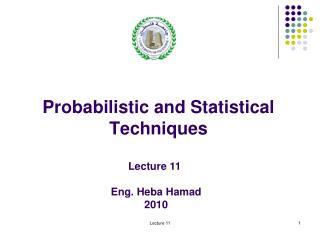DownloadDownload PresentationProbabilistic and Statistical Techniques

# Probabilistic and Statistical Techniques

Télécharger la présentation## Probabilistic and Statistical Techniques

- - - - - - - - - - - - - - - - - - - - - - - - - - - E N D - - - - - - - - - - - - - - - - - - - - - - - - - - -
##### Presentation Transcript

1. Probabilistic and Statistical Techniques Lecture 11Eng. Heba Hamad2010 Lecture 11

2. Probabilistic and Statistical Techniques Example Lecture 11

3. Probabilistic and Statistical Techniques Lecture 11

4. Probabilistic and Statistical Techniques Lecture 11

5. Probabilistic and Statistical Techniques Lecture 11

6. Probabilistic and Statistical Techniques Event Event C D Total 4 2 6 A 1 3 4 B 5 5 10 Total Example What’s the Probability? • P(A) = • P(D) = • P(CB) = • P(AD) = • P(BD) = Lecture 11

7. Probabilistic and Statistical Techniques Event Event C D Total 4 2 6 A 1 3 4 B 5 5 10 Total Solution The Probabilities Are: • P(A) = 6/10 • P(D) = 5/10 • P(CB) = 1/10 • P(AD) = 9/10 • P(BD) = 3/10 Lecture 11

8. Probabilistic and Statistical Techniques Event Probability Using Two–Way Table Event Event B B Total 1 2 P(A ) A P(A  B ) P(A  B ) 1 1 1 1 1 2 A P(A ) P(A  B ) P(A  B ) 2 2 1 2 2 2 P(B ) P(B ) 1 Total 1 2 Lecture 11

9. Probabilistic and Statistical Techniques Color Type Total Red Black 2 2 4 Ace 24 48 Non-Ace 24 26 52 26 Total Conditional Probability Using Two–Way Table Experiment: Draw 1 Card. Note Kind, Color & Suit. Lecture 11

10. Probabilistic and Statistical Techniques Event Event C D Total 4 2 6 A 1 3 4 B 5 5 10 Total Example Using the table then the formula, what’s the probability? • P(A|D) = • P(C|B) = • Are C & B Independent? Lecture 11

11. Probabilistic and Statistical Techniques Solution Using the formula, the probabilities Are: P(A  D) 2 / 10 2 P(A | D) = = = P(D) 5 / 10 5 P(C  B) 1 / 10 1 P(C | B) = = = P(B) 4 / 10 4 5 1 ≠ = P(C | B) P(C) = Dependent 10 4 Lecture 11

12. Probabilistic and Statistical Techniques Example Suppose that we have two events, A and B with P(A)=0.5, P(B)=0.6 and P(A &B)=0.4 Find: P(A/B) P(B/A) Are A and B independent events? Why? Lecture 11

13. Probabilistic and Statistical Techniques Example Assume that we have two events, A and B that are disjoints, assume also that P(A)=0.3, P(B)=0.4 What is (A & B) What is P(A & B) What is P(A/B) Lecture 11

14. Probabilistic and Statistical Techniques From a very large sample Lecture 11

15. Probabilistic and Statistical Techniques From a very large sample • P (Died of Cancer) = 0.00739 • P (Died of Cancer/ Current Cigar Smoker) = .00103/ .057= 0.018 • Since P (Died of Cancer) does not equalP (Died of Cancer/ Current Cigar Smoker) • So the events are dependent Lecture 11

16. Probabilistic and Statistical Techniques Example In a survey of MBA students, the following data were obtained on ‘students’ first reason for application to the school in which they joined. Lecture 11

17. Probabilistic and Statistical Techniques Lecture 11

18. Probabilistic and Statistical Techniques Example • The probability that a bomber hits its target on any particular mission is 0.8 . Four bombers are sent after the same target. What is the probability: - they all hit the target - none hit the target - at least one hits the target Lecture 11

19. Probabilistic and Statistical Techniques • What can you say about: - P(A B) + P(A B’) = - P(A B) + P(B A’) =     Lecture 11

20. Probabilistic and Statistical Techniques • A bag contains 200 balls that are red or blue and either dull or shiny. There are 55 shiny red balls, 91 shiny balls, and 70 red balls. If a ball is chosen at random, what is the probability that it is either a shiny ball or a red ball ? Lecture 11

21. Probabilistic and Statistical Techniques • In how many ways can six people sit in six seats in a line ? 6! • In how many ways can the six people sit around a dinner table eating pizza ? 5! ways Lecture 11

22. For 3 letters: There is 3! =6 Possible arrangements But if they are arranged in a circular manner, just 2!= 2 arrangements will appear. A A C B Lecture 11

23. For 4 letters: There is 4! =24 Possible arrangements But if they are arranged in a circular manner, just 3!= 6 arrangements will appear. A A D B C Lecture 11